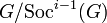Socle series

This article defines a quotient-iterated series with respect to the following subgroup-defining function: socle

Definition

Suppose$G$ is a group. The socle series of$G$ is an ascending series of subgroups$\operatorname{Soc}^i(G)$,$i \in \mathbb{N}$, defined as follows:

•$\operatorname{Soc}^0(G)$ is the trivial subgroup of$G$.
• For$i > 0$,$\operatorname{Soc}^i(G)$ is the unique subgroup of$G$ such that$\operatorname{Soc}^i(G)/\operatorname{Soc}^{i-1}(G)$ is the socle of$G/\operatorname{Soc}^{i-1}(G)$.

The series can be extended to a transfinite series. In that case, the definition is as follows for ordinals:

• For any limit ordinal$\alpha$,$\operatorname{Soc}^\alpha(G)$ is the union of$\operatorname{Soc}^\beta(G)$,$\beta < \alpha$.
• For$\beta + 1$ the successor ordinal to$\beta$,$\operatorname{Soc}^{\beta + 1}(G)/\operatorname{Soc}^\beta(G)$ is the socle of$G/\operatorname{Soc}^\beta(G)$.

Since socle is strictly characteristic, the socle series is a strictly characteristic series, i.e., all members of the series are strictly characteristic subgroups of$G$.

For a finite p-group

For a finite p-group, and more generally, for a nilpotent p-group,$\operatorname{Soc}(G)$ coincides with$\Omega_1(Z(G))$ (see socle equals Omega-1 of center in nilpotent p-group). The socle series in this case is also called the upper exponent-p central series, and is the fastest ascending exponent-p central series. The corresponding fastest descending exponent-p central series is the lower exponent-p central series.